Tutorials
data visualization
+2

Shareable Data Science with Kyso

In this tutorial, you’ll learn how to create publishable and reproducible data science studies on Kyso’s platform, using interactive plotly visualizations.

We've spent the last few months designing a system to improve collaboration, reproducibility, and presentation - an all-inclusive tool that optimizes the entire workflow of a data scientist. We've spoken to hundreds of data scientists over the last year gathering feedback, and we are now excited to bring you the latest version of Kyso.

Think of it like Github, but specifically for data science.

The result is a tool to run, publish and share Jupyter notebooks, somewhere you can build upon the courses and projects completed with Datacamp and create your own data science portfolio. It's a free tool to showcase and share your work, get feedback and find cool & interesting new projects.

For a more comprehensive guide, check out this announcement, Introducing Kyso 2.0, recently posted upon our latest release. For now, here is a quick summary of what the platform offers:

• Free Jupyterlab workspaces to start and run notebooks.
• Blog-style rendering of these notebooks with the option to show or hide your code.
• A custom Jupyterlab extension that allows users to publish to their Kyso profile from any Jupyterlab environment.
• A profile page very much suited to building and hosting your data science portfolio.
• Simple discovery for finding cool new projects to fork onto your own workspaces.
• And many more features coming soon!
import pandas as pd
import numpy as np

import plotly.plotly as py
from plotly.offline import init_notebook_mode, iplot
init_notebook_mode(connected=True)
import plotly.graph_objs as go
from plotly import tools

from IPython.display import Image

I felt the best way to demonstrate the mechanics and purpose of the platform would be to actually publish this example study with some cool and interesting data visualizations. These plots are generated using plotly and & are completely interactive when rendered on Kyso. You can rotate the globes, zoom in on specific areas & highlight data points.

I've simply embedded screenshots of the plots for this post on DataCamp but check out the live notebook published here:

KyleOS | datacamp-intro

I've uploaded two cool datasets just to play around with - however, there is so much more depth to the data than what I've plotted below. Sign up for free, fork this study (along with the attached data files) onto your own Jupyterlab environment, extend the analysis & come up with some cool visualizations yourself. When you're ready, you can simply re-publish!

Modern Slavery

The Global Slavery Index publishes a report each year with information on modern slavery, which applies to various factors that make people vulnerable like forced labor, human trafficking, etc.., as well as government responses and products in the global supply chains that are at risk of being produced by modern slavery.

colors=[[0, '#380000'], [0.05, '#500000'],
[0.15, '#680000'], [0.2, '#800000'],
[0.25, '#980000'], [0.35, '#A80000'],
[0.45, '#B80000 '], [0.55, '#C00000'], [1.0, '#FF0000']]

plotmap = [ dict(
type = 'choropleth',
locations = df['Country '],
locationmode = 'country names',
z = df['Est. number of people in modern slavery'],
text = df['Country '],
colorscale = colors,
reversescale = False,
marker = dict(
line = dict (
color = 'rgb(180,180,180)',
width = 0.5
) ),
colorbar = dict(
title = ""),
) ]

layout = dict(
title = "Estimated number of people in modern slavery worldwide",
geo = dict(
showframe = False,
showcoastlines = False,
projection = dict(
type = 'natural earth'
)
),
height=700,
width=900
)

fig = dict( data=plotmap, layout=layout )
iplot(fig)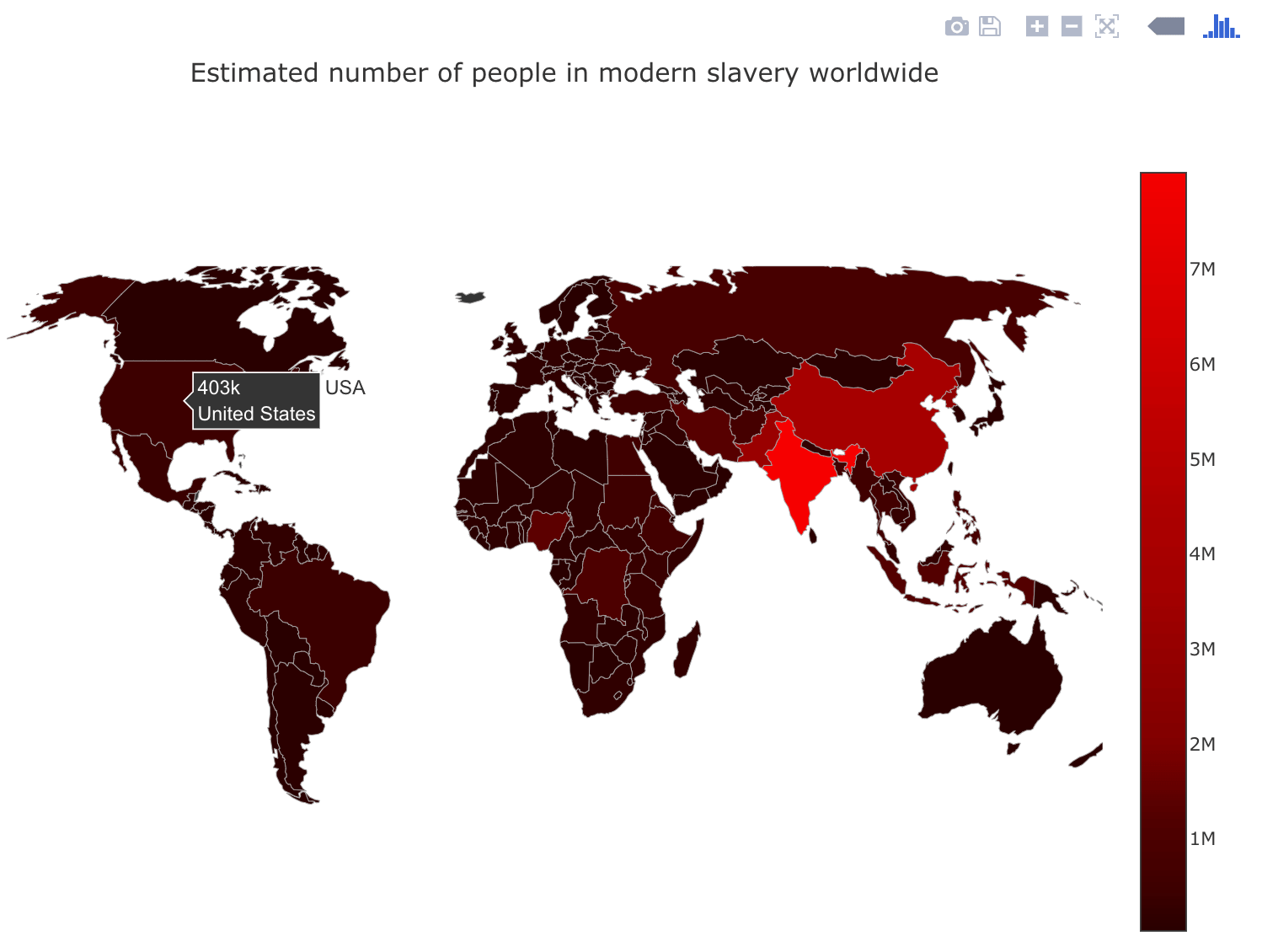Maybe an idea to improve on the above map here would be to plot out the prevalence of modern slavery in each country, meaning the numbers expressed above as percentages of national populations.

The Global Slavery index Vulnerability Model maps 23 risk variables across five major dimensions, and assigns a score to each country's dimension based on these variables. One of the five dimensions that naturally has a part to play is Inequality - so let's map out the levels of global inequality.

colors=[[0, 'rgb(102,194,165)'], [0.05, 'rgb(102,194,165)'],
[0.15, 'rgb(171,221,164)'], [0.2, 'rgb(230,245,152)'],
[0.25, 'rgb(255,255,191)'], [0.35, 'rgb(254,224,139)'],
[0.45, 'rgb(253,174,97)'], [0.55, 'rgb(213,62,79)'], [1.0, 'rgb(158,1,66)']]

plotmap = [ dict(
type = 'choropleth',
locations = df['Country '],
locationmode = 'country names',
z = df['Factor Three Inequality'],
text = df['Country '],
colorscale = colors,
reversescale = False,
marker = dict(
line = dict (
color = 'rgb(180,180,180)',
width = 0.5
) ),
colorbar = dict(
title = ""),
) ]

layout = dict(
title = "Global Inequality",
geo = dict(
showframe = False,
showcoastlines = False,
showocean = True,
oceancolor = '#26466D',
projection = dict(
type = 'orthographic'
)
),
height=700,
width=900
)

fig = dict( data=plotmap, layout= layout )
iplot(fig)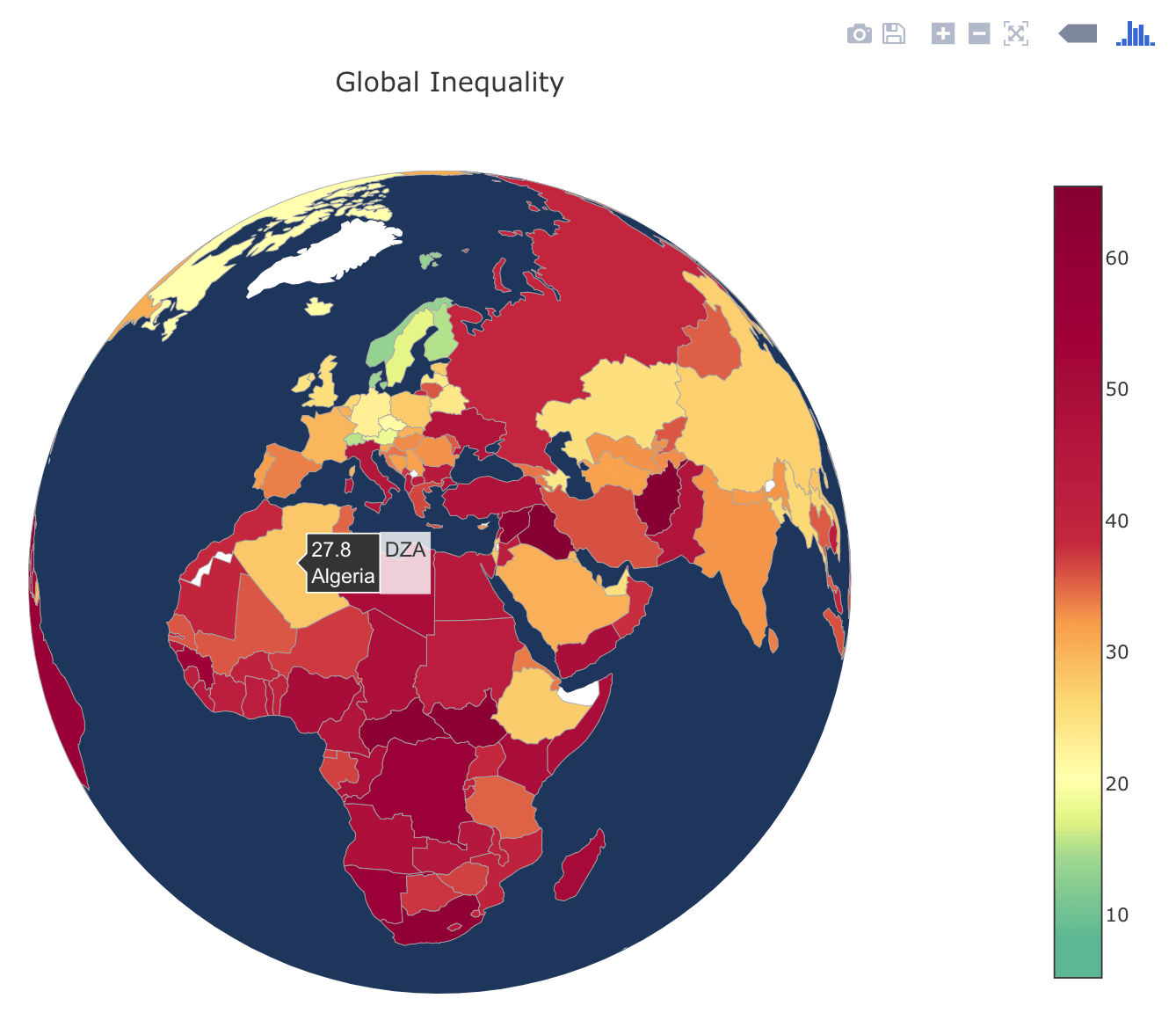The World's Religions

The World Religion Project aims to provide detailed information about religious adherence worldwide since 1945 and is hosted by Zeev Maoz, University of California-Davis, and Errol A. Henderson, Pennsylvania State University. It contains data about the number of adherents by religion in each of the states in the international system.

df = df[df['year'] == 2010]
data = [ dict(
type = 'choropleth',
autocolorscale = False,
colorscale = 'Greens',
reversescale = True,
showscale = True,
locations = df['state'].values,
z = (df['christianity_all'].values/df['population'].values)*100,
locationmode = 'country names',
text = df['state'].values,
marker = dict(
line = dict(color = 'rgb(200,200,200)', width = 0.5)),
colorbar = dict(autotick = True, tickprefix = '',
title = '%')
)
]
layout = dict(
title = 'Christian Adherents in 2010 as Percentage of Population',
geo = dict(
showframe = True,
showocean = True,
oceancolor = '#26466D',
projection = dict(
type = 'orthographic',
rotation = dict(
lon = 60,
lat = 10),
),
lonaxis =  dict(
showgrid = False,
gridcolor = 'rgb(102, 102, 102)'
),
lataxis = dict(
showgrid = False,
gridcolor = 'rgb(102, 102, 102)'
)
),
height=700,
width=900
)

fig = dict(data=data, layout=layout)
iplot(fig, validate=False)

plotmap = [ dict(
type = 'choropleth',
locations = df['state'].values,
locationmode = 'country names',
z = (df['islam_all'].values/df['population'].values)*100,
text = df['state'].values,
colorscale = 'Viridis',
reversescale = False,
marker = dict(
line = dict (
color = 'rgb(180,180,180)',
width = 0.5
) ),
colorbar = dict(
title = "%"),
) ]

layout = dict(
title = "Islam Adherents in 2010 as Percentage of Population",
geo = dict(
showframe = False,
showcoastlines = False,
projection = dict(
type = 'natural earth'
)
),
height=700,
width=900
)

fig = dict( data=plotmap, layout=layout )
iplot(fig)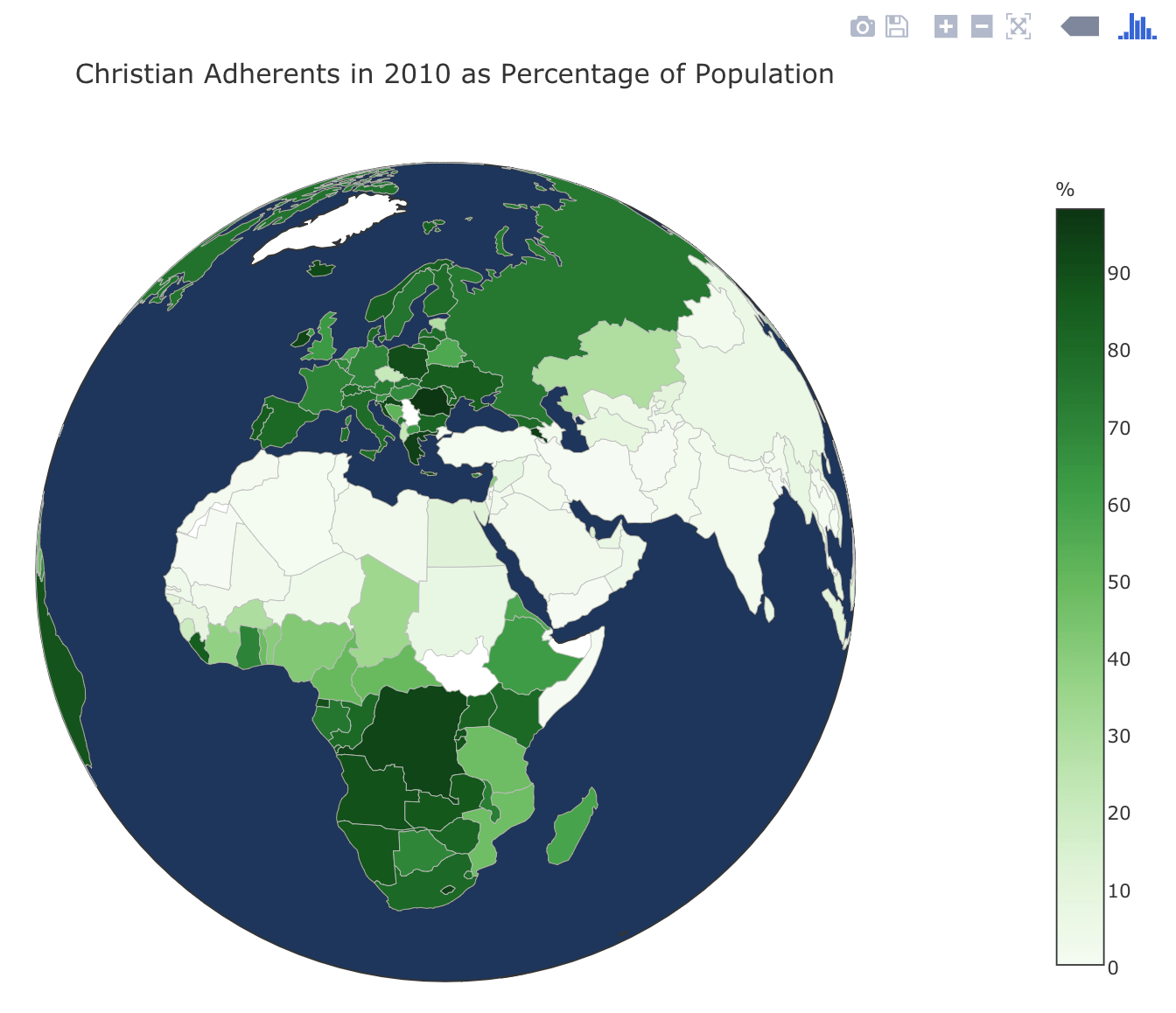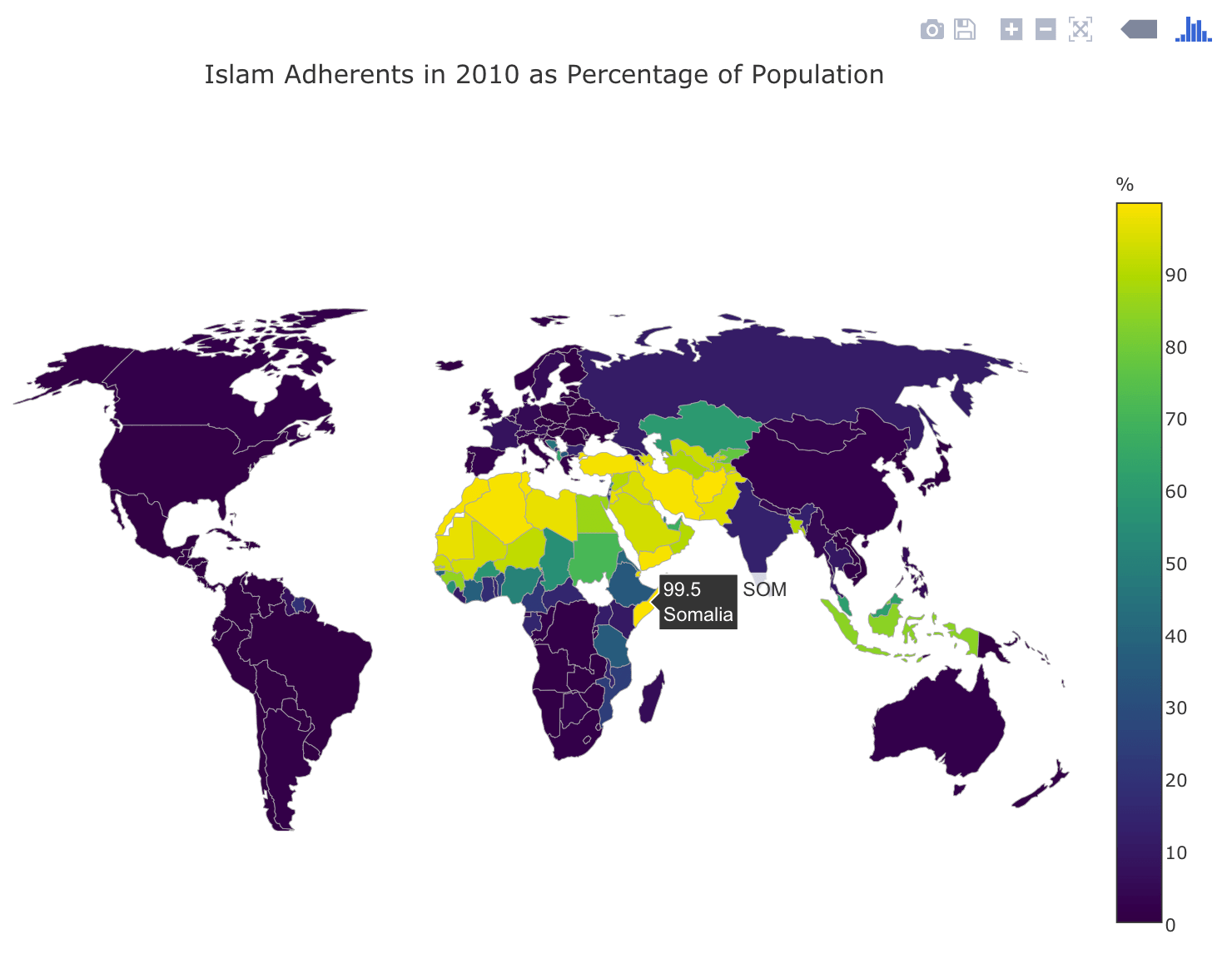Pretty cool! How about generating a time series of the total number of adherents to all religions evolving over time? I imagine we will see an overall decline in religiosity.

df_usa = df[df['code'] == 'USA']
df_usa = df_usa.set_index('year')

df_ire = df[df['code'] == 'IRE']
df_ire = df_ire.set_index('year')

df_gmy = df[df['code'] == 'GMY']
df_gmy = df_gmy.set_index('year')

df_uk = df[df['code'] == 'UKG']
df_uk = df_uk.set_index('year')

df_spn = df[df['code'] == 'SPN']
df_spn = df_spn.set_index('year')
trace1 = go.Scatter(
x = df_usa.index,
y = df_usa['noreligion_percent'],
mode = 'lines+markers',
name = 'USA',
marker=dict(color='#90EE90')
)

trace2 = go.Scatter(
x = df_ire.index,
y = df_ire['noreligion_percent'],
mode = 'lines+markers',
name = 'Ireland',
marker=dict(color='#008744')
)

trace3 = go.Scatter(
x = df_gmy.index,
y = df_gmy['noreligion_percent'],
mode = 'lines+markers',
name = 'Germany',
marker=dict(color='rgb(12, 12, 140)')
)

trace4 = go.Scatter(
x = df_uk.index,
y = df_uk['noreligion_percent'],
mode = 'lines+markers',
name = 'United Kingdom',
marker=dict(color='#851e3e')
)

trace5 = go.Scatter(
x = df_spn.index,
y = df_spn['noreligion_percent'],
mode = 'lines+markers',
name = 'Spain',
marker=dict(color='#FFA505')
)

data = [trace1, trace2, trace3, trace4, trace5]

layout = go.Layout(title="Increase in Percentage of Population with No Religion",
height=500,
xaxis={'title':'Year',
'showgrid':False},
#                   plot_bgcolor='rgb(245,245,240)',
#                   paper_bgcolor='rgb(245,245,240)',
yaxis={'title':'Percentage of Population with no Religion',
'showgrid':False,
'tickformat': ',.0%'})

fig = go.Figure(data=data,layout=layout)

iplot(fig)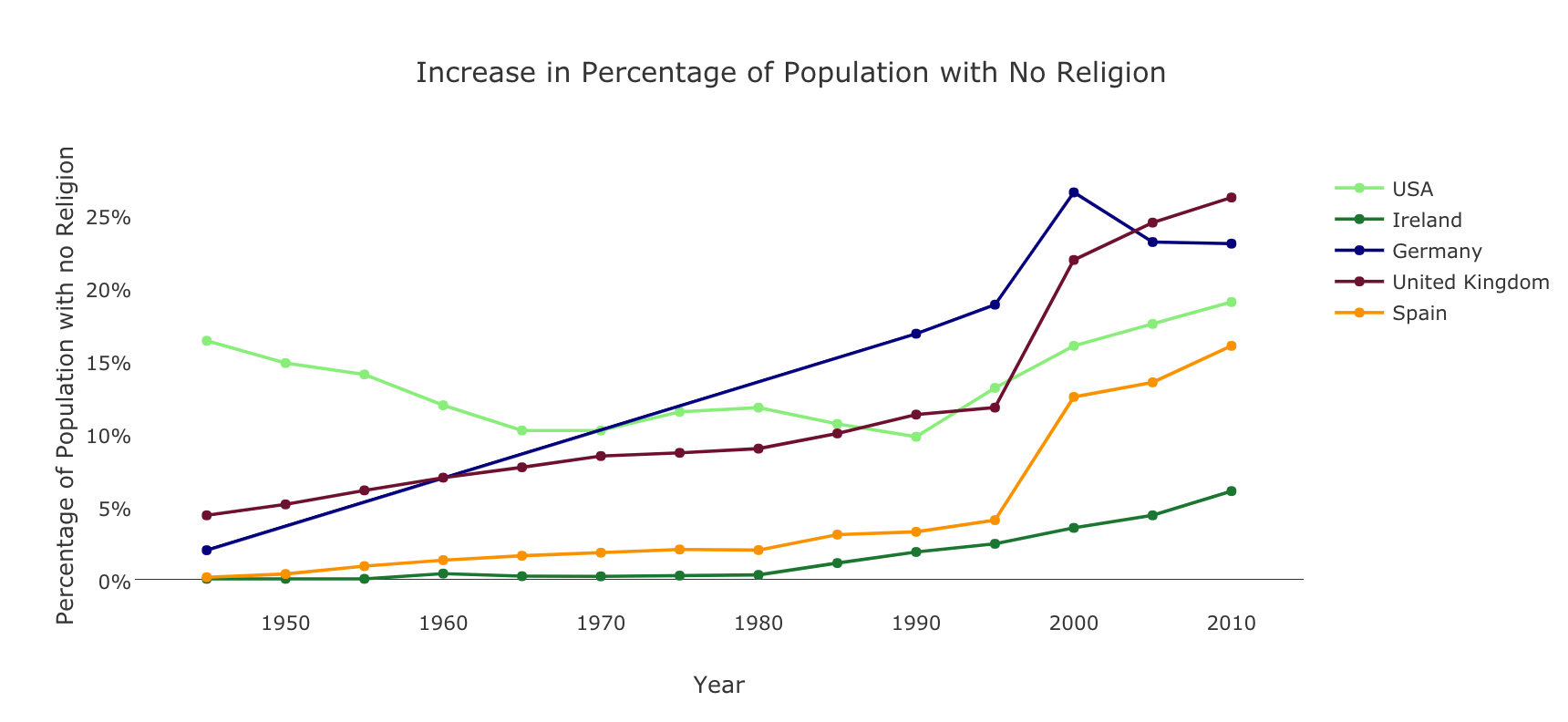That's it for this brief post guys. There are, however, over 30 columns in the first dataset and over 70 in the second, meaning there is the possibility of much deeper analysis. If you're new to plotly, there is a quick-fire guide here. Our explore page also contains content most recently published if you'd like to discover other projects.

Try out the platform - feel free to reach out with feedback and/or ideas for future features - Kyso 2.0 is in beta & we take the feedback from our users very seriously. Don't hesitate to contact me directly at [email protected]

Happy Coding!